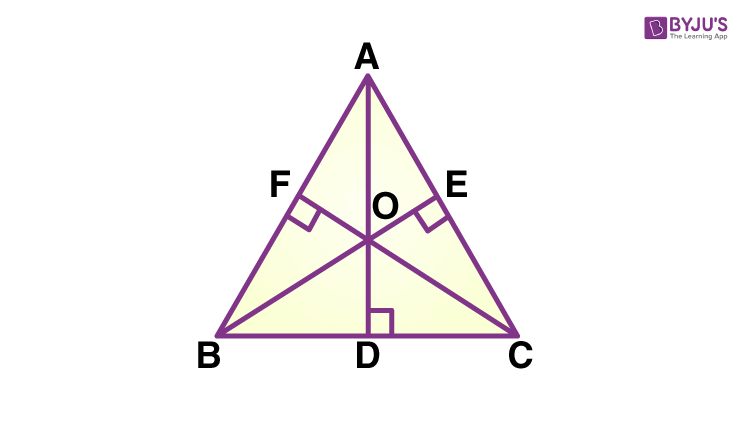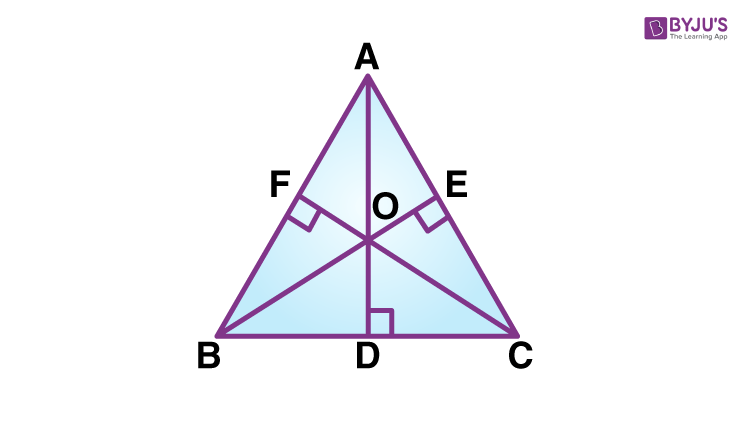# Orthocenter

The orthocenter is the point where all the three altitudes of the triangle cut or intersect each other. Here, the altitude is the line drawn from the vertex of the triangle and is perpendicular to the opposite side. Since the triangle has three vertices and three sides, therefore there are three altitudes. Also learn, Circumcenter of a Triangle here.

The orthocenter will vary for different types of triangles such as Isosceles, Equilateral, Scalene, right-angled, etc. In the case of an equilateral triangle, the centroid will be the orthocenter. But in the case of other triangles, the position will be different. Orthocenter doesn’t need to lie inside the triangle only, in case of an obtuse triangle, it lies outside of the triangle.

## Orthocenter of a Triangle

The orthocenter of a triangle is the point where the perpendicular drawn from the vertices to the opposite sides of the triangle intersect each other.

• For an acute angle triangle, the orthocenter lies inside the triangle.
• For the obtuse angle triangle, the orthocenter lies outside the triangle.
• For a right triangle, the orthocenter lies on the vertex of the right angle.

Take an example of a triangle ABC.In the above figure, you can see, the perpendiculars AD, BE and CF drawn from vertex A, B and C to the opposite sides BC, AC and AB, respectively, intersect each other at a single point O. This point is the orthocenter of △ABC.

## Orthocenter Formula

The formula of orthocenter is used to find its coordinates. Let us consider a triangle ABC, as shown in the above diagram, where AD, BE and CF are the perpendiculars drawn from the vertices A(x1,y1), B(x2,y2) and C(x3,y3), respectively. O is the intersection point of the three altitudes.

First, we need to calculate the slope of the sides of the triangle, by the formula:

m = y2-y1/x2-x1

Now, the slope of the altitudes of the triangle ABC will be the perpendicular slope of the line.

Perpendicular slope of line = -1/Slope of the line = -1/m

Let slope of AC is given by mAC. Hence,

mAC = y3-y1/x3-x1

Similarly, mBC = (y3-y2)/(x3-x2)

Now, the slope of the respective altitudes are:

Slope of BE, mBE = -1/mAC

Now here we will be using slope point form equation os a straight line to find the equations of the lines, coinciding with BE and AD.

Therefore,

mBE = (y-y2)/(x-x2)

Hence, we will get two equations here which can be solved easily. Thus, the value of x and y will give the coordinates of the orthocenter.

Also, go through Orthocenter Formula

## Properties of Orthocenter

The orthocenter is the intersection point of the altitudes drawn from the vertices of the triangle to the opposite sides.

• For an acute triangle, it lies inside the triangle.
• For an obtuse triangle, it lies outside of the triangle.
• For a right-angled triangle, it lies on the vertex of the right angle.
• The product of the parts into which the orthocenter divides an altitude is the equivalent for all 3 perpendiculars.

## Construction of Orthocenter

To construct the orthocenter of a triangle, there is no particular formula but we have to get the coordinates of the vertices of the triangle. Suppose we have a triangle ABC and we need to find the orthocenter of it. Then follow the below-given steps;

• The first thing we have to do is find the slope of the side BC, using the slope formula, which is, m = y2-y1/x2-x1
• The slope of the line AD is the perpendicular slope of BC.
• Now, from the point, A and slope of the line AD, write the straight-line equation using the point-slope formula which is; y2-y1 = m (x2-x1)
• Again find the slope of side AC using the slope formula.
• The perpendicular slope of AC is the slope of the line BE.
• Now, from the point, B and slope of the line BE, write the straight-line equation using the point-slope formula which is; y-y1 = m (x-x1)
• Now, we have got two equations for straight lines which is AD and BE.
• Extend both the lines to find the intersection point.
• The point where AD and BE meets is the orthocenter.Note: If we are able to find the slopes of the two sides of the triangle then we can find the orthocenter and its not necessary to find the slope for the third side also.

## Orthocenter Examples

Question:

Find the orthocenter of a triangle whose vertices are A (-5, 3), B (1, 7), C (7, -5).

Solution:

Let us solve the problem with the steps given in the above section;

1. Slope of the side AB = y2-y1/x2-x1 = 7-3/1+5=4/6=⅔

2. The perpendicular slope of AB = -3/2

3. With point C(7, -5) and slope of CF = -3/2, the equation of CF is y – y1 = m (x – x1) (point-slope form)

4. Substitute the values in the above formula.

(y + 5) = -3/2(x – 7)

2(y + 5) = -3(x – 7)

2y + 10 = -3x + 21

3x + 2y = 11 ………………………………….(1)

5. Slope of side BC = y2-y1/x2-x1 = (-5-7)/(7-1) = -12/6=-2

6. The perpendicular slope of BC = ½

7. Now, the equation of line AD is y – y1 = m (x – x1) (point-slope form)

(y-3) = ½(x+5)

Solving the equation we get,

x-2y = -11…………………………………………(2)

8. Now when we solve equations 1 and 2, we get the x and y values.

Which are, x = 0 and y = 11/2 = 5.5

Therefore(0, 5.5) are the coordinates of the orthocenter of the triangle.

Try out: Orthocenter Calculator

Download the BYJU’S App and get personalized video content to experience an innovative method of learning.

## Frequently Asked Questions – FAQs

Q1

### What is orthocenter?

The orthocenter is the point where the altitudes drawn from the vertices of a triangle intersects each other.
Q2

### What is the difference between orthocenter and circumcenter?

The orthocenter is the point of intersection of three altitudes drawn from the vertices of a triangle.
The circumcenter is the point of intersection of the perpendicular bisector of the three sides.
Q3

### Where does the orthocenter of the obtuse triangle lie?

The orthocenter of the obtuse triangle lies outside the triangle.
Q4

### Where does the orthocenter of right triangle lie?

The orthocenter of a right-angled triangle lies on the vertex of the right angle.
Q5

### What is the difference between orthocenter and centroid?

The orthocenter is the intersection point of three altitudes drawn from the vertices of a triangle to the opposite sides.
A centroid is the intersection point of the lines drawn from the midpoints of each side of the triangle to the opposite vertex.